Do you like the article?
Share it with others -
Use new possibilities of MetaTrader 5

# A scientific approach to the development of trading algorithms

7 December 2020, 09:05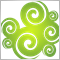2
3 548

### Introduction

Financial market trading without a proper trading system has all the chances to ruin the trader's deposit. No matter which markets is selected - the long-term outcome would be the same for an inexperienced trader. A trading system or an algorithm is required for generating profits.

There are a lot of different trading systems and algorithms, including a separate category of mathematically profitable algorithms, such as arbitrage strategies, HFT, options strategies and SPOT symbol trading against derivatives. However, all well-known algorithms have their disadvantages. They either have low profitability or require high competition for liquidity and order execution speed. That is why traders try to develop their own algorithms based on unique logic.

As a rule, most of trading algorithms utilize asset price analysis in an effort to forecast future prices with some probability. Other algorithms use not only price analysis, but also external data, as well as take into account fundamental and news background, including the analysis of rumors.

The problem is that very few people understand exactly how to look for patterns, which are efficient and which are not, and why. Why, having attached standard indicators or any other found indicators to a chart, they cannot get a profitable algorithm. They try to optimize strategy parameters in the optimizer in an effort to generate a profit, but they actually simply adjust strategy parameters to a small section of history, and then suffer losses in a forward period. The optimizer is a necessary and useful tool, but it should be used to find optimal parameters for a profitable algorithm and not to try to make a profitable system out of a losing one by adjusting parameters on historical data.

Unlike other areas, trading develops poorly due to the high competition. Profitable and disruptive ideas are usually kept secret and are not discussed online. While unprofitable ideas or ideas that do not generate profit spread very quickly. This is because if a person or a group of people develop something really worthwhile, they do not need to share their developments with others - they monetize on their knowledge. If they unveil the basic of their system, this will create competitors who will also try to grab liquidity, which is far from infinite. Therefore, everyone who comes to algorithmic trading has to collect any information from scratch and gain their own experience. It can take years to understand the basic rules.

Because of all these factors, there are more superstitions in trading than really working rules. When it comes for the scientific approach, it is the same in all spheres, allowing you to progress faster when developing your profitable algorithms. Therefore, let us consider how to adhere to a scientific approach and avoid superstitions during the development of a trading algorithm. We will consider some ideas in this article using a simple trading system as an example.

### Search for pricing patterns

The development of a trading algorithm should begin with a search for a pricing pattern which will provide a positive math expectation of profit during trading. The pattern can stem from a previously developed pricing hypothesis or can be found by chance. Both options often happen in science: some inventions are made by chance, while other are the product of long-term research. The earlier article "Price series discretization, random component and noise" considered the usage of block chart and described the reasons for their usage. So, I will use block charts instead of candlestick charts.

In my previous article entitled "What is a trend and is the market structure based on trend and flat?", I have developed a definition for the concept of a trend and conducted a study of whether the market structure is based on trend or flat. I recommend reading this article so that you can understand further ideas.

The analysis has shown that most markets have a trend structure, which means that the probability of a trend continuation is higher than the probability of a reversal. This follows from the fact that the increments distribution density in N steps for price series is wider and lower than that for a process with a 50% probability of a reversal at each step. An example is shown in figure 1.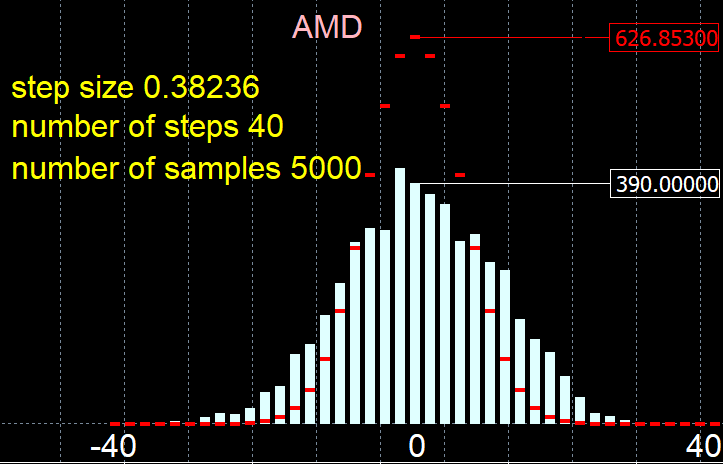Figure 1.

In this figure, red shows the reference distribution of the increments probability density for a process of 40 steps, in which the probability of a reversal at each next step is equal to 50%. 5000 samples were used here. The white histogram shows the measured values for 40 steps of AMD stock (again, for 5000 samples). The number of vertical steps and the number of passes by the price in 40 steps was calculated for 5000 measurements; the result was displayed as a histogram.

In other words, 5000 segments of 40 steps were used to calculate how many steps each of the segments passed vertically, and the result was displayed as a histogram. If we take an example of AMD stocks (40 steps), the price passed 0 vertical steps 390 times; for the reference the price must pass 626.85 times.

This chart shows that the market price passed zero vertical steps in 40 steps much less frequently than a process in which the probability of a reversal at each next step is 50%. Therefore, the analyzed chart has a trend continuation probability above 50%, and thus the stock is likely to continue the trend. In other words, having passed 10 points the price is more likely to move another 10 points in the same direction than to reverse.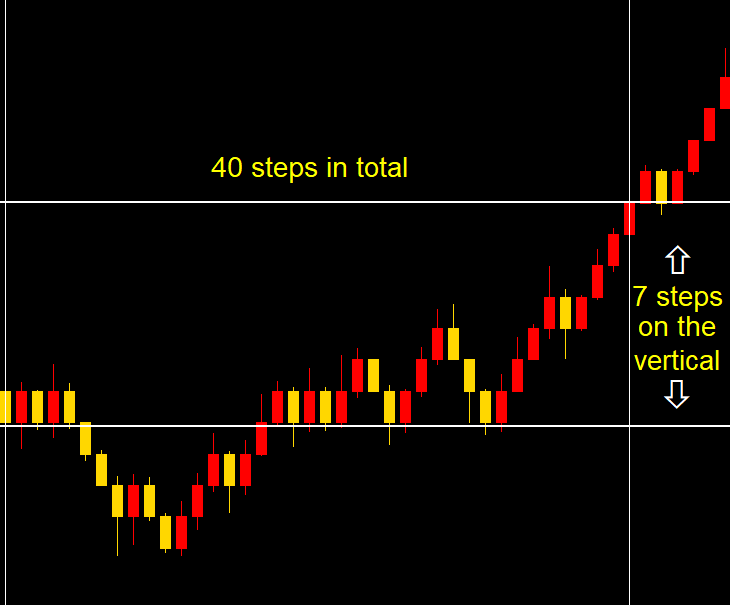Image 2.

Figure 2 shows an example of how the number of vertical steps was counted. Here, we calculate how many blocks the price has passed vertically for these 40 blocks (steps). In the above figure, the price passed 7 vertical steps in 40 steps. Then we analyze another 4999 samples 40 steps each, and measure their vertical movement. The measured results are displayed as a histogram on which the X axis shows an amplitude and the Y axis shows the number of amplitude hits.

### Analysis of the found pattern

Once a pattern is found, it needs to be analyzed. Take other trading symbols and block size scales in order to understand if the found pattern is only typical for this specific trading instrument with a specific file, or if it applies to all trading instruments at any scale. In this example, I have analyzed 30 currency pairs, a lot of stocks traded in the Russian and US markets, a dozen of major cryptocurrencies and commodity symbols. All scales with the block size from one point to thousands of points were used for each symbol. According to the analysis conclusion, all trading instruments tend to have a trend-based structure in any scale, but the trendy nature weakens as the scale increases.

The following patterns have been detected: markets are likely to be trending on any scale, but the trend strength weakens on higher scales. It means that after passing N points vertically, there is a probability of more than 50% that the price will move the same distance in the same direction. The advantage of this pattern is that it allows using a simple trend strategy for trading, where you can open a Buy position after each upward step and open a Sell position after each downward step.

After that the applied approach is analyzed. Perhaps this is not the pattern that we have found, but an error in the market structure analysis method. In this case, markets are not trending and we cannot generate profit from the above assumption. We need to start with the basics and understand how the block, in which we found trends, are built. You can use the block indicator presented in the previous article entitle "What is a trend and is the market structure based on trend and flat?". In this case, the price series is quantized ("sliced") in blocks N points each. A new block of N points is formed every time when the price passes N points from the previous reference point. If the block size is 10 points, the price moves 10 points and forms another block having open and close prices. Then wait until the price moves another 10 points in one direction and close another block, and so on. This seems easy at first glance, but blocks are built using the closing prices of 1-minute candlesticks - this will be important later.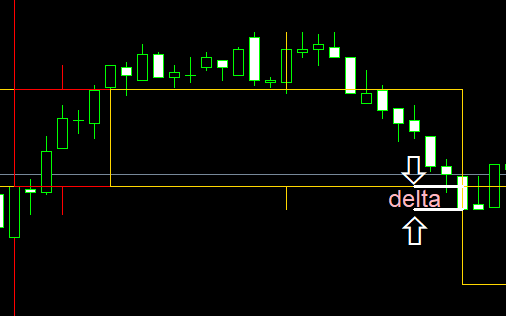Image 3.

Further analysis reveals 2 problems:

1. Delta between the candlestick Close and the block Close. Figure 3 shows a situation when the closing price of a falling block is higher than the closing price of a falling candlestick. The delta between the block Close and the candlestick Close can spoil all the profit that we planned to generate from exploiting the ideal of a trend. The reason is connected with our intention to use a simple algorithm: open Buy positions after each growing block and open Sell after each falling block. Since blocks are formed at candlestick close, the signal of a formed block will only be generated after the candlestick closes. Due to this, the position will be opened at a worse price. This delta can eat up all the profits and the expected payoff will be zero.
2. Commissions and spread. Perhaps the market trend is only enough to compensate for spread and commissions. In this case, if we open/close positions by market, losses from opening at a worse price and commissions will be greater than all the profits that can be generated using this pattern. Well, we can use limit orders in exchange markets to eliminate spreads, but we still have to pay commission. Furthermore, limit orders do not eliminate spreads in the forex market.

Thus, two problems have been determined, which can prevent you from generating a profit out of the detected pattern. The following can assist in understanding the influence of the problems on the resulting profit:

• Measure the probability of trend continuation for a specific instrument on a large sample - this allows obtaining the exact probability value for calculating the expected payoff.
• Collect point-statistics for the delta between the block Close and the expected position Open price and find the average value.
• Find the average spread when working with market orders or when trading forex symbols and add commissions.
• Subtract all the calculated overhead costs in deposit currency from the resulting expected payoff and get a forecast for the system's real expected payoff.
• If the resulting expected payoff remains above zero, we can proceed to the next step.

It would be correct to execute all the above steps, but a more interesting solution is to write a simple trading robot and to check this in practice. At least, it would be more interesting to view the operation results of a ready-made algorithm in this article. There can be other specific features which we have missed. Now, assume that we have calculated everything, have received a positive result and wish to create a trading algorithm based on this pattern. So, let us move on to the next step.

### Developing a hypothesis explaining the presence of this pattern

It is dangerous to use the found pattern without having a hypothesis that explains its nature. We still do not know why the pattern exists and if it is stable. We do not know when it will disappear and when it will appear again. When investing real money in trading, it is necessary to be able to track the presence of a pattern and to understand when the deposit has a prolonged drawdown from its maximum and will recover soon, and when the pattern has disappeared, and it is time to stop the robot. It is exactly the moment that distinguishes a profitable robot from a loss-making one. That is why it is necessary to develop a hypothesis that explains the presence of this pattern. This hypothesis can be further transformed into a theory and used for adjusting the algorithm operation modes.

The pattern exists in all analyzed instruments and in all scales, but the trendiness decreases on larger scales. Probably, the two factors mentioned above (delta and commission) really affect this pattern. Therefore, let us put forward two theses:

• The larger the block size, the smaller the relative delta during block close and the less error when opening/closing a position. This is because all blocks are based on 1-minute candlestick close prices, and their size is fairly stable in a certain range. Accordingly, the larger the block size and the smaller the delta, the less trend the instrument tend to have. Thus, the error in trend determining method is reduced.
• The larger the block size, the smaller the influence of spread and commissions on market characteristics because spread and commission size becomes small relative to the movement size. Since the market is quite efficient, it may react to this fact by proportionally decreasing the trend.

A small note here: even if we eliminate delta errors on a small scale, the markets are indeed trending, but their trend is only enough to compensate for the spread and commissions. Developers of scalping algorithms often make mistakes because they do not know this fact. What they think: "I need to find a broker with lower commissions and my scalping robot will start generating profit. It will show great results if I can reduce the spread and commissions." But it will not, because the market trend on smaller scale can only cover spread and commission. If the spread and commissions were smaller, then other market participants would have already used this pattern and destroyed it. This does not refer to all scalpers - I only mean simple trading robots which do not use front running, Market Depth volume analysis and other working techniques.

Suppose not only the analyzed but also all other markets are based on trend. But why are all the markets based on trend?

If you do not understand pricing on exchange markets, I recommend that you first read the article entitled "Principles of Exchange Pricing through the Example of Moscow Exchange's Derivatives Market". If you do know the basics, it will be easier for you to understand the idea. Actually, it does not matter which market you trade, exchange, crypto or forex. The fundamentals of pricing are the same everywhere. However, trading conditions and analysis possibilities can be different.

For simplicity, let us consider the boundary options for the price series shape. Two opposite scenarios are possible for a price chart: a linear infinite trend and a sinusoid. A sinusoid would be convenient, as everyone would know when to buy and sell an asset. A linear ascending chart would also be convenient: obviously you need to constantly buy in order to generate profits. But such chart forms are impossible, because there will be no buyers at the highs, and there will be no sellers at the lows. Figure 1 shows a hypothetical example of a situation when the price chart is sinusoidal, and an appropriate Depth of Market.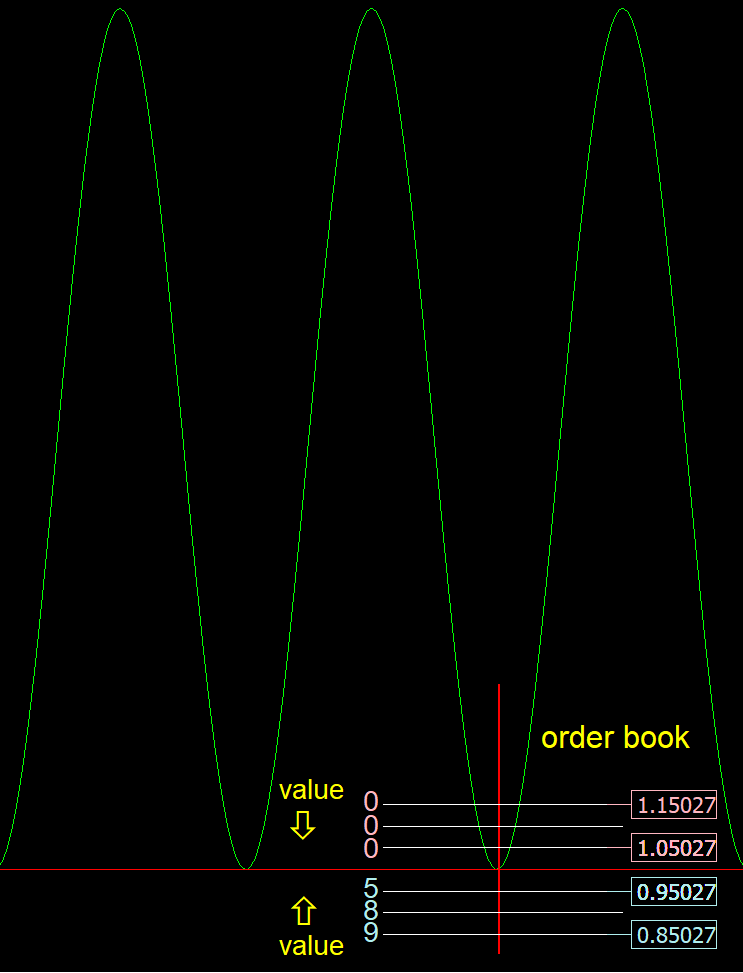Figure 4.

So, if the price chart is sinusoidal, then there will be no people willing to sell the asset at the Market Depth low, because everyone knows that the price will not go lower. On the contrary, everyone will want to buy such an asset at its minimum price. Since there will be no people willing to sell the asset, no deals will be executed, and the price will not be able to move along such a trajectory. The market will start searching an equilibrium price, which will be suitable for buyers to buy and for sellers to sell.

A similar situation will occur for the linearly ascending chart. Since everyone knows that the asset price is growing all the time, no one will sell it, and if no one sells the asset, then no one can buy. This means that such a price chart is also impossible. So, buyers must buy, and sellers must sell in order for a price chart to exist. There must be someone who makes a mistake when determining the benefit. However, since each participant seeks to maximize profit and does not want to make mistakes, the chart should be more complex than a sinusoid and more complex than a linear ascending chart.

The price chart in an efficient market should be somewhere in the middle, between the linear and sinusoid charts. Its structure must be complex enough, so that the buyer and seller profit is not obvious. Sinusoidal and linear charts are characterized by low entropy. The entropy must be greater to provide for the possibility to execute deals. The more participants in the market and the "smarter" they are, the stronger the price chart will tend to maximum entropy.

If you consider Shannon's entropy, then it takes its maximum value on a uniform distribution. The market process is not uniform but is more like a normal one. However, a normal distribution can be obtained from a uniform one and vice versa. Moreover, we use blocks with a fixed step size. In other words, the maximum entropy is the characteristic of a process that has no regularities, in which the direction change probability at each next movement is 50%. But our analysis shows that the direction change probability for the market chart is different from 50%, which means there is memory and the entropy is not maximum.

The market will strive for maximum entropy, but this state will be reached only when there is an infinite number of participants (very high liquidity) or when the participants are infinitely "smart". "Smart" here refers to the ability to determine complex patterns. "Smarter" participants can identify more complex and less obvious patterns. An infinitely "smart" participant can identify and exploit absolutely all patterns. The condition (either infinite number of participants, or infinitely smart participants) is used because an infinite number of participants will have infinite computational ability, and they will be able to identify all the patterns using brute force, even if they are not very "smart".

This hypothesis explains why financial instrument price charts are becoming more complex. At the beginning of the 20th century, one could profit by simply using an MA. Now, as algo-trading develops, participants become "smarter", patterns become more complex, entropy grows, and it becomes more difficult to make money in the market. What does "become smarter" mean? They have greater computing power, faster decision-making, the ability to determine their benefits faster and more accurately, and the ability to find more complex patterns.

But we have found quite a simple pattern. This can be explained by a constant inflow of capital in the market. Market participants do not take all the profits, and a trend component appears. The capital inflow is connected with the money creation. Each country constantly issues its own currency, maintaining a certain level of inflation. This money issuance probably creates the trend component.

The following conclusions can be made from the hypothesis explaining the pattern:

• Continuous emission of money causes the market to become trending. In this case, actively developing assets with high investment attractiveness should have a stronger trend structure than non-developing assets and the markets that have weak investment attractiveness.
• The symbol trend strength will decrease over time, because the number of participants grows, the participants become "smarter", and the asset cannot develop indefinitely. The asset rate of development and attractiveness decrease over time, though each asset is different.
• Developed currency pairs (such as EURUSD) should have lower trend strength than emerging stocks because popular currency pairs attract more participants who make more trades, which increases entropy. Moreover, currencies are traded by banks who have to conduct foreign exchange operations and have large resources to create "smart" trading algorithms in order to generate additional profit. Furthermore, currency pairs such as EURUSD have very high liquidity, and high liquidity leads to a decrease in trend.

### Hypothesis testing

In the previous section "Developing a hypothesis explaining the presence of this pattern" we only put forward a hypothesis in an effort to explain the existence of a pattern. The hypothesis is not 100% correct and may contain inaccuracies, because it is only a step in an algorithm development process. This is an example of how you can develop logical reasoning while explaining a pattern. This approach is used to avoid market superstitions as much as possible. For example, if we had a pattern like: "If RSI (Stochastic / MACD / Elliott waves / your variant) enters the overbought zone and forms a pattern, then sell", then you should ask yourself: "Why should this work?" and "What are the fundamental reasons for this to work?"

We will test the hypothesis roughly, without going deep into details, while we need understand whether the conclusions correlate with real data. We can compare the trendiness degree of different instruments. According to the assumption, actively developing and investment attractive trading instruments should have stronger trendiness than developed ones or than less investment-attractive instruments. According to the second assumption, the more participants trade an asset, the higher the liquidity, and the less trending it will be. Let us conduct a simple check of this assumption.

At the end of the article "What is a trend and is the market structure based on trend and flat?", we compared the trendiness degree of several assets, including EURUSD, AMD, AAPL and others. Let us perform a similar comparison using 4 symbols as an example:

• The EURUSD currency pair as an asset attracting the largest number of participants and thus having low investment attractiveness and high liquidity. Currencies have a low investment attractiveness because there is inflation: money always depreciates and investing in a currency is unprofitable in the long term.
• Apple stocks (AAPL) as the most attractive and developing investment instrument, which is traded by a large number of private individuals. The stock has high liquidity, but it is significantly less than that of EURUSD.
• Sberbank (SBER) stocks have significantly lower liquidity, lower investment attractiveness on a global scale than that of Appl, and the number of participants is correspondingly lower.
• AMEZ stocks. They have very low investment attractiveness and very low liquidity.Figure 5a.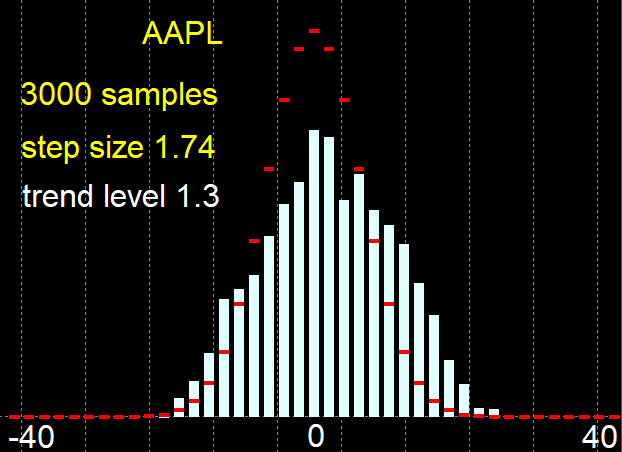Figure 5b.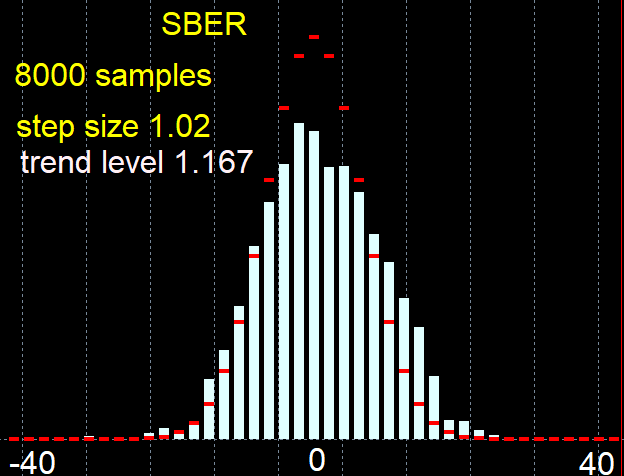Figure 5c.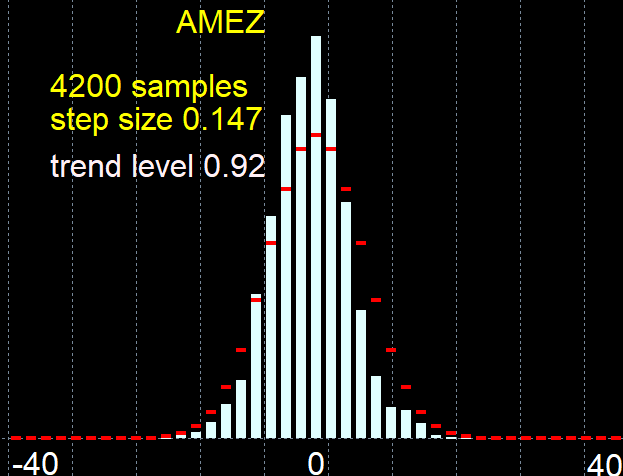Figure 5d.

As can be seen from Figures 5, EURUSD has a low degree of trendiness = 1.068, Appl stock has high trendiness = 1.3, Sberbank has trendiness of 1.168, i.e. lower than that of Appl, but higher than that of EURUSD. AMEZ stocks have a flat structure, and their trend degree is below 1.

The following conclusion can be made: the assumption that high investment attractiveness increases the degree of trendiness, and high liquidity and a large number of participants reduce the degree of trade, does not contradict the experimental data, which means that the hypothesis can be used in the future.

Let us develop a trading algorithm for the found pattern. The pattern is simple, therefore the trading algorithm will also be simple. Trading will be based on blocks, which means the M1 candlestick charts should be converted to blocks N points each. The block building algorithm will be integrated into the trading robot. The trading algorithm will follow directly from the formula for determining the expected payoff:

```m=(P(tp)*tp)-(P(sl)*sl)

```

where:

• m  math expected payoff,
• P(tp)  the probability of a profitable trade,
• P(sl)  the probability of a losing trade,
• tp  average profitable trade size,
• sl — average losing trade size.

It will work as follows:

1. Open a position after another falling or growing block has closed;
• if the block was falling, open a Sell position;
• if the block was rising, open a Buy position;
• After opening a position, wait for a closing signal.
2. Close the position when a block in the opposite direction forms. If a Buy position is open, wait for a falling block to form and close the position. After a position is closed on a falling block, a Sell position can be opened. Thus there will be only one position in the market.
3. Figure 3 shows the case when a candlestick and a block close at different prices and a delta is formed. This is connected to the specific feature of the block formation algorithm. So, we need price control to avoid opening at an unfavorable price. Let us add another condition: if the delta between the candlestick close price and the block close price is greater than a certain value, a position should not be opened - wait for the price to return to the close price of the block and then open a position.
4. Add placing of Take Profit and Stop Loss. They are needed id the candlestick size turns out to be significantly larger than the block size. Thus, profitable/unprofitable positions will be closed not only when the block is closed, but also on large spikes;
• SL price is calculated as follows: block size (BS) is multiplied by a coefficient from the settings (ksl); for Sell positions: add the result Sl=Bclose(op)+BS*ksl to the close price of the block on which the position opening signal was generated (Bclose(op)). For Buy positions: subtract Sl=Bclose(op)-BS*ksl from the block close price;
• TP price is calculated as follows: block size (BS) is multiplied by a coefficient from the settings (ktp); for Sell positions: subtract the result TP=Bclose(0)-BS*ktp from the close price of the last block (Bclose(0)). For Buy positions: add Tp=Bclose(0)+BS*ktp to the last block close price. In contrast to Stop Loss, here we use the close price of each new block and recalculate Take Profit after closure of each new block. This will help earn additional profit when candlesticks significantly larger than the block size appear.
5. Lot management function. In addition to standard lot management functions, let us add a function that will change lot size in accordance with the current block size. This will allow preserving stable risk level and profit when the block size grows. Let us introduce the concept of the minimum block size (minimum BS), relative to which the lot size will be adjusted. Position opening lot is a lot from the settings divided by the ratio of the current block size to the minimum size from the settings: lot = lot(set)/(BS/minimum BS).
6. Determining the block size. This function is very important for the stable operation of the algorithm. There are several possible options:
• fixed block size specified in the settings in the price format, for example 0.02;
• automatic block size, which will be linked to the size of the candlesticks of the current working timeframe. The candlestick size can be obtained from the ATR indicator. Then multiply the current ATR value by the coefficient to obtain the block size. For example, we want to trade blocks sized 5*ATR. So with an increase in volatility, the block size will grow and the system will adapt to changing market parameters. You can use ATR from the current working timeframe or from a higher timeframe if you want to experiment.
7. Permission to open Buy/Sell positions. Let us add a function that allows opening Buy and Sell positions separately. This will enable a separate analysis of Buy and Sell signals.

The resulting robot is attached below. This robot has some more functionality, but we will not consider it in this article.

The operation of the algorithm will be tested on those assets whose behavior we analyzed. Previously, we predicted on which assets the algorithm should hypothetically be profitable. Now, let us check how the algorithm works on these assets in order to check the correctness of the hypothesis.

Let us test using EURUSD. The symbol has a weak trend and, perhaps, this may not be enough to cover commissions and delta. The final algorithm operation is shown in Figures 6a and 6b.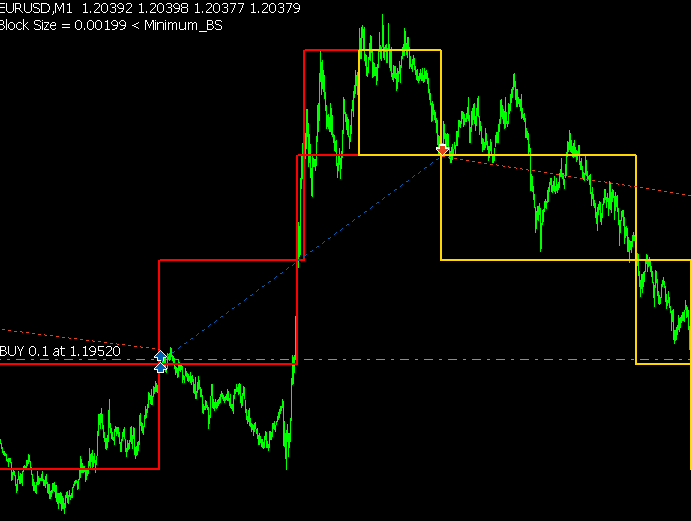Figure 6a.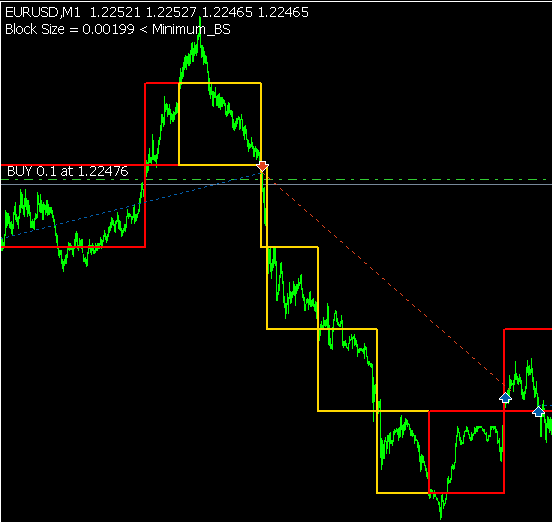Figure 6b.

Testing was performed in the period from 01.01.2018 to 28.07.2020, on the M1 timeframe, using the real tick mode. Parameters were not optimized, because I want to show that there can be no need to optimize a thoroughly prepared algorithm for each individual currency pair. We will change block size, minimum block size and lot, in an effort to have profit significantly exceeding commission size.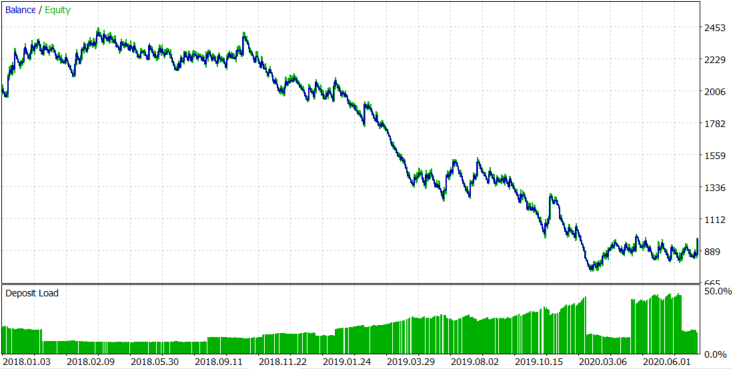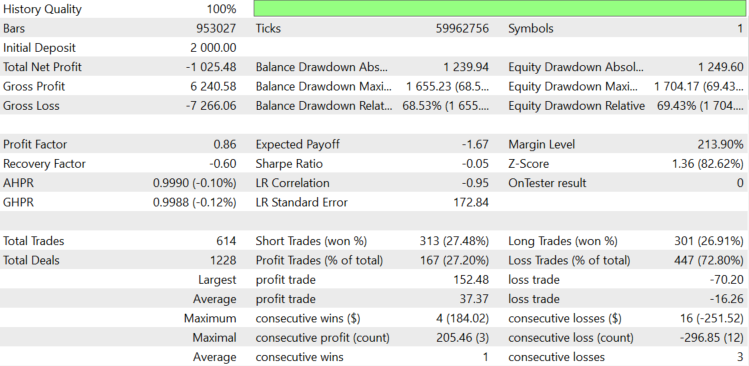Figure 7.

For EURUSD, as expected, spread and delta took all the profit that we should have received from the asset trend. As a result, the expected payoff us -\$1.67 per trade. The lot was changed dynamically, depending on the block size, with the average lot being 0.078. Let us try to understand where the loss comes from. The robot logs information about the spread. The average spread during position opening and closing is 0.00008. We paid swaps of \$159.76, and opened 614 positions. So, the average swap per position was 159.76/614=\$0.2602.

If the average spread is 0.00008 and the average lot is 0.078, 1 EURUSD pip with a lot of 0.078 is equal to \$0.078, and so spread costs 0.078*8=\$0.624. In total, the commission is equal to \$0.624+\$0.2602=\$1.104. If we were losing a commission on each deal, the expected payoff would be -\$1.104, but it is \$1.67, which is \$0.566 more. The minimum block size is set to 0.002 in settings, so it makes \$15.6 for an average lot of 0.078. Let us roughly estimate the negative balance change if the balance chart were a random walk and the block size was always minimal. It is calculated as 15,6*(614^0.5)=386.55\$. Now, add the average commission per trade multiplied by the number of trades. 1.104*614+386.55=\$1064.406.

The value is equal to \$1064.406, which means the average drawdown of a balance chart if the probability of position opening in the right direction is 50% and a commission is paid for each open position. In reality, we received a loss of \$1027.45, which is close to this value. We can conclude that we had a loss, because the expected payoff of our algorithm is zero for EURUSD.

Let us see the results on the more trending AAPL stocks. The result is shown in Figure 8 below.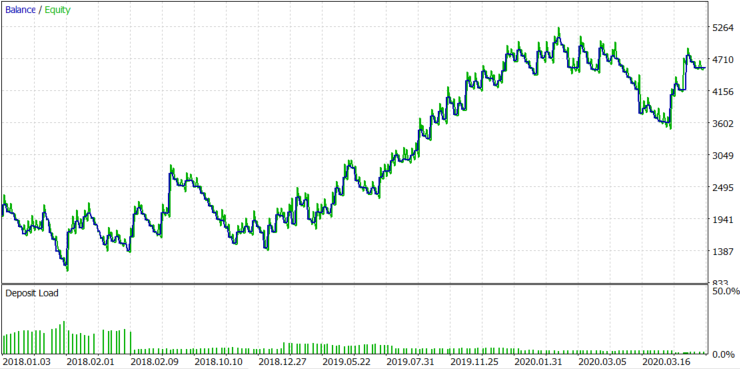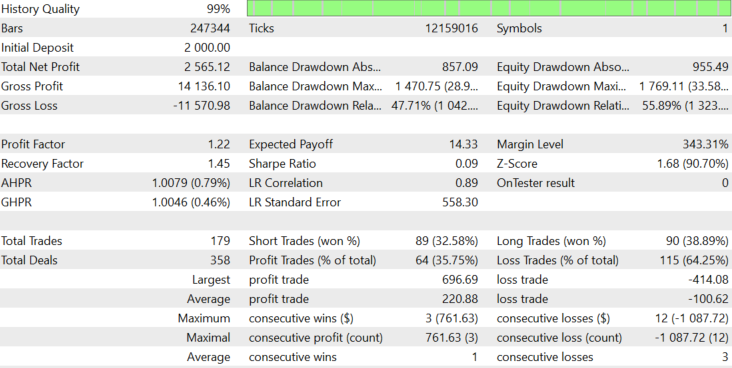Figure 8.

The result is stably positive, with the expected payoff equal to 19.33. This was a test with a dynamic lot (set in accordance with the block size), and the block size changed dynamically with the volatility. Let us see what happens if we don't change the lot dynamically. The average lot size in Figure 8 was 39. Let us use the fixed lot of 39 and check the result in figure 9.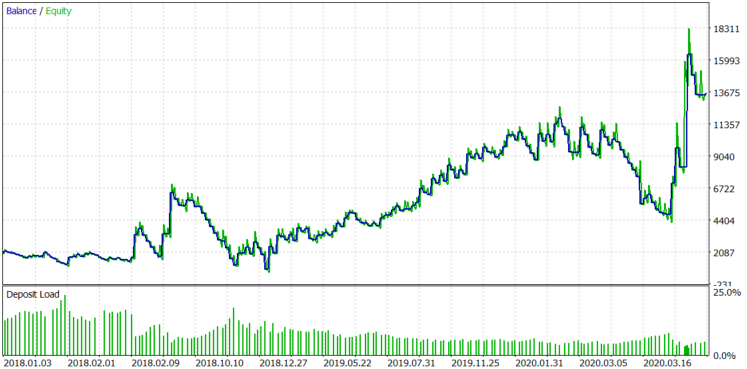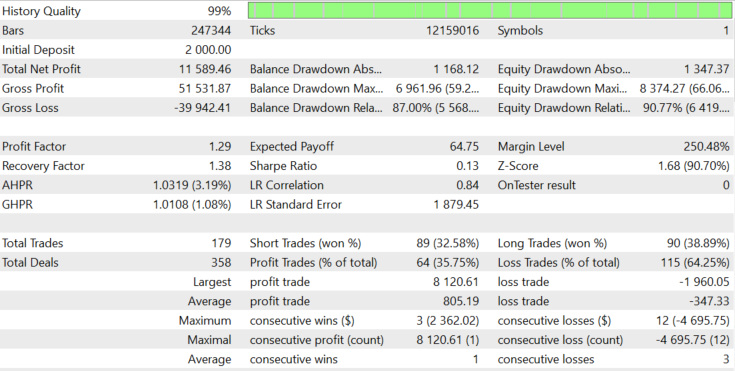Figure 9.

The result has not changed much, with the exception of the last profitable trade and some increased balance drawdowns. The block size on APPL stocks grows over time, and a larger block with a fixed lot can show larger profit, but the probability to drawdowns can increase proportionally.

Next, let us see what happens if only Sell positions are allowed. The results are shown in Figure 10.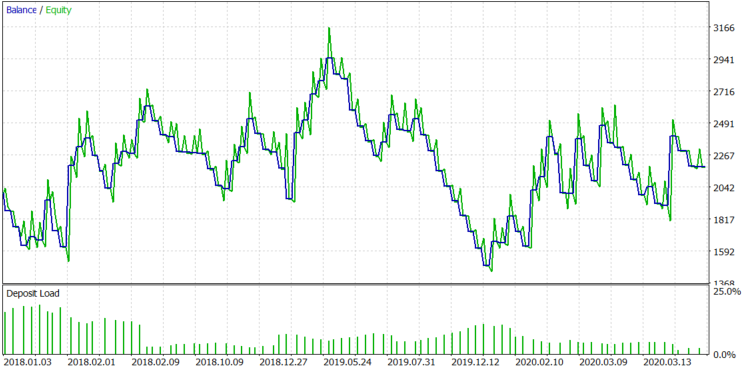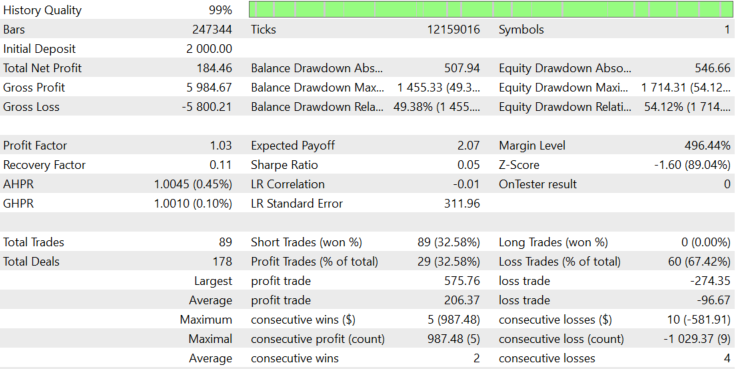Figure 10.

The Sell-only mode shows a small loss, but Figure 5b shows the asymmetry of the distribution relative to zero, so this result could be expected. Next, let us check Buy-only mode. The result is shown in figure 11.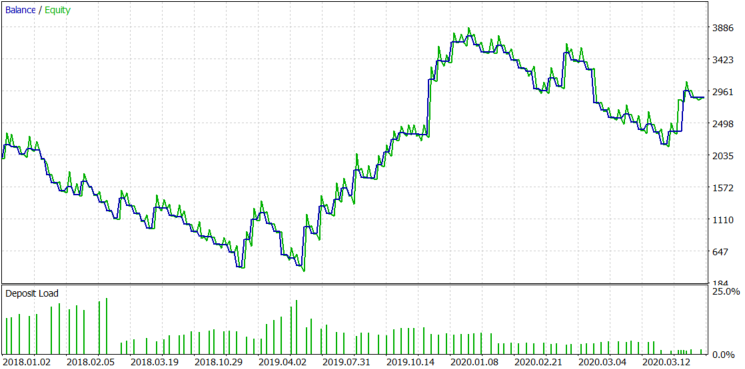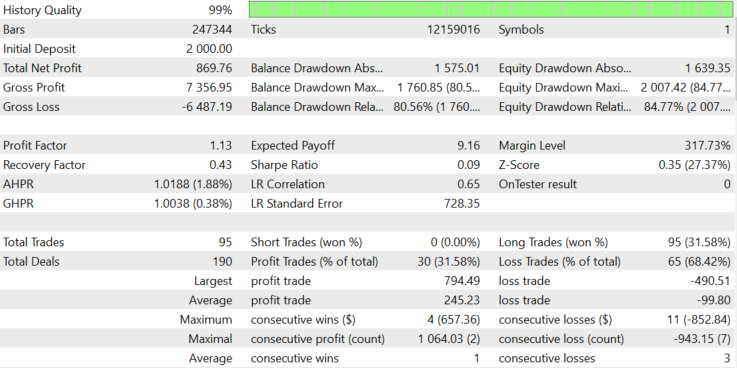Figure 11.

As you can see from Figure 11, the Buy-only mode shows positive results, but not so good as Buy and Sell mode. Since the stock has alternating upward and downward trend sections, the use of both Buy and Sell positions makes the balance graph more even.

Let us check how the algorithm works on other actively developing company stocks. Let us start with AMD. Testing will be performed in the period from 2018.06.01 to 2020.07.28, because the broker provides 1-minute history starting with this date. This test is performed using dynamic lot with Buy and Sell positions. The results are shown in Figure 12.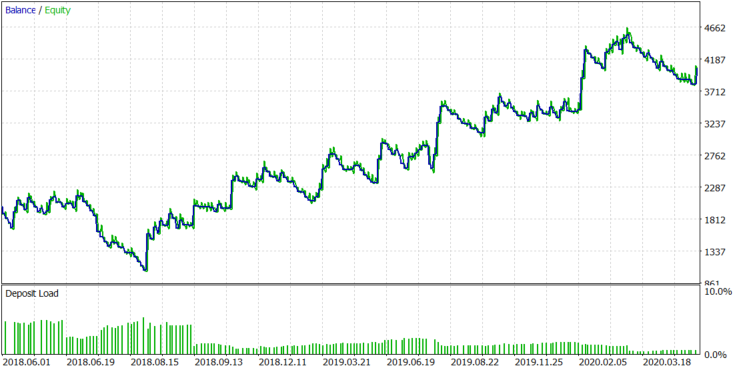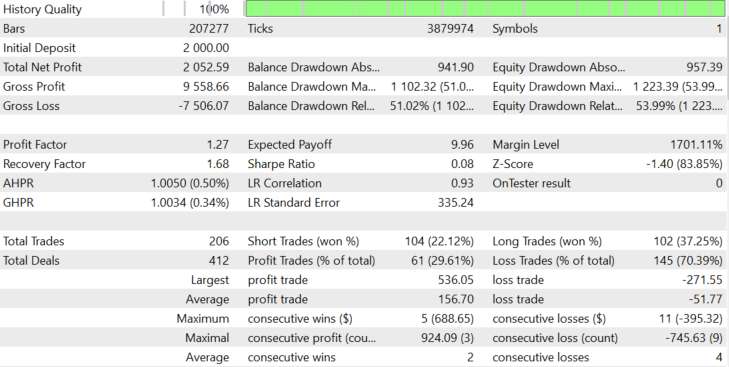Figure 12.

With AMD stocks, the algorithm shows a stable positive result, which matches the assumptions made on the basis of the distribution shown in Figure 1. Now, let us check another actively developing company, Tesla. The algorithm is shown in Figure 13.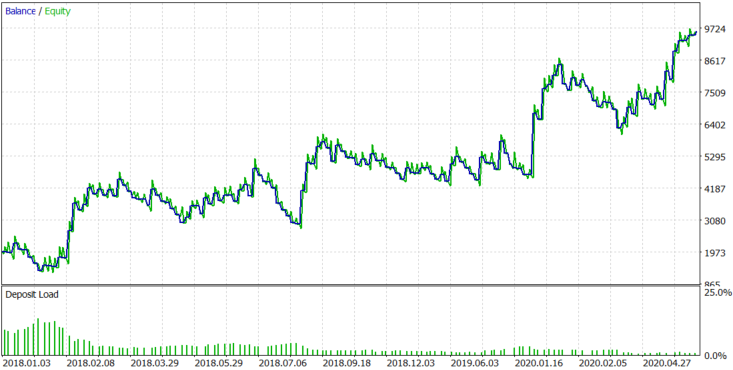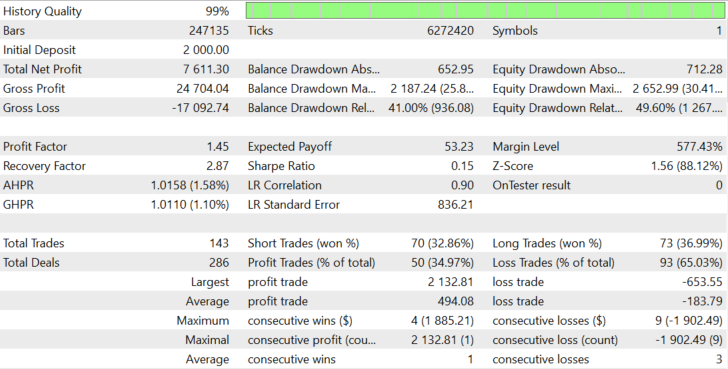Figure 13.

The algorithm shows stable results with TESLA stocks because this company, like the previous ones, has high investment attractiveness and great growth potential.

For comparison, let us test the algorithm with Sberbank shares, which is one of the leading Russian companies. The same testing period is shown. Commissions are equal to the ones offered by the Otkrytie broker = 0.057% per volume. Testing was performed without leverage, using real tick mode. The test result is shown in Figure 14.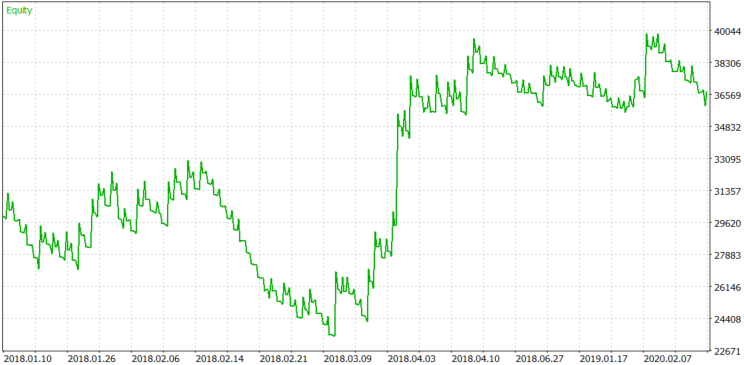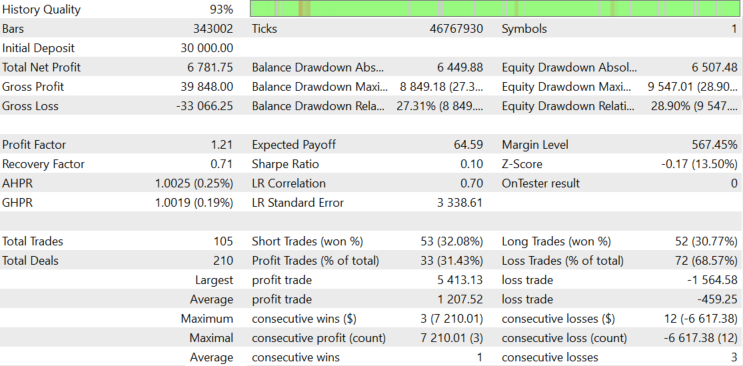Figure 14.

Although Sberbank is not as attractive as the above assets, the stock is still trending using such a simple algorithm, because of low liquidity.

All settings and testing reports are attached below, so you can analyze them in detail.

### Conclusion

The following conclusions can be made by analyzing the behavior of the created trading algorithm:

• The found pattern allowed us to create an algorithm that is capable of generating profit without the need to optimize parameters for each trading instrument. Parameters are changed manually, but we know in advance why we set this or that parameter.
• Knowing the mechanism for changing the parameters, we can make a fully automatic algorithm.
• We clearly understand which pattern we use to generate a profit, and thus we can learn to predict the pattern strengthening or weakening in the future.
• The presence of a pattern on a particular instrument can be predicted by using a hypothesis.
• The market trend emergence hypothesis should be developed and turned into a full-fledged theory describing the laws of pricing. By developing the theory, we can improve the stability, reliability and versatility of the algorithm.
• As a specific trading instrument develops further, the pattern will become less vivid. In this case a good solution is to switch to new actively developing instruments.
• The trading method can be profitable, but it requires improvement. It is necessary to reduce the delta between block close and position open price to a minimum - this delta occurs because of block formation specifics. One of the solutions is to switch to the analysis of tick charts, instead of minute ones.
• Knowing the average trending level of an instrument, it is possible to collect statistics on trendiness degree change over time and approximate it using non-linear equations. Further, knowing the trendiness change laws, it is possible to develop a mechanism for controlling the deviation from the average trendiness in order to increase the profitability of the system.
• This algorithm in its current form is very simple; it was only used to test the idea, but the idea has shown its viability and potential. So, the algorithm can be significantly modified and used in real markets.
• It is easier to generate profit in the stock market than in forex. One of the reasons is that stock market assets are more trendy. But there are also flat instruments, such as the above example with AMEZ. The algorithm can be modified for trading on such instruments, to make it work not expecting the continuation of the trend, but a reversal. But this is a topic for a separate study, because assets with low investment attractiveness may have liquidity problems.
• The algorithm executes deals by market; the logic can be changed to trade limit orders in an effort to increase profitability. This will complicate the logic but can increase profitability. Anyway more research and development are needed.
• The article has shown a practical method of applying the concept of trendiness, which was described in my previous article entitled What is a trend and is the market structure based on trend and flat? and the practical application of switching from candlestick charts to block charts described in the article

#### Price series discretization, random component and noise.

The author of the idea and the development is Maxim Romanov, the Expert Advisor code is written by Sergey Chalyshev according to the provided previously terms of reference.

Translated from Russian by MetaQuotes Software Corp.
Original article: https://www.mql5.com/ru/articles/8231

Attached files |
report.zip (706.34 KB)
Set.zip (6.23 KB)
robot.zip (13.03 KB)
Last comments | Go to discussion (2)| 8 Dec 2020 at 09:33
MetaQuotes:

New article A scientific approach to the development of trading algorithms has been published:

Author: Maxim Romanov

Thanks for the interesting article. There is an custom indicator missing: Max_distribution_v.1.13.mq5 Can you please upload it? Thanks.| 8 Dec 2020 at 09:48
konorti:
Thanks for the interesting article. There is an custom indicator missing: Max_distribution_v.1.13.mq5 Can you please upload it? Thanks.

Thank you for appreciating the article. Max Distribution V.1.13.mq5 indicator I did not attach it to the article, because it is a unique, author's development and I am not ready to exhibit it for general use. <Deleted>  I warn you right away, for the robot laid out in the article, it is of no value.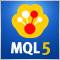CatBoost machine learning algorithm from Yandex with no Python or R knowledge required

The article provides the code and the description of the main stages of the machine learning process using a specific example. To obtain the model, you do not need Python or R knowledge. Furthermore, basic MQL5 knowledge is enough — this is exactly my level. Therefore, I hope that the article will serve as a good tutorial for a broad audience, assisting those interested in evaluating machine learning capabilities and in implementing them in their programs.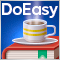Timeseries in DoEasy library (part 50): Multi-period multi-symbol standard indicators with a shift

In the article, let’s improve library methods for correct display of multi-symbol multi-period standard indicators, which lines are displayed on the current symbol chart with a shift set in the settings. As well, let’s put things in order in methods of work with standard indicators and remove the redundant code to the library area in the final indicator program.Timeseries in DoEasy library (part 51): Composite multi-period multi-symbol standard indicators

In the article, complete development of objects of multi-period multi-symbol standard indicators. Using Ichimoku Kinko Hyo standard indicator example, analyze creation of compound custom indicators which have auxiliary drawn buffers for displaying data on the chart.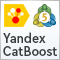Advanced resampling and selection of CatBoost models by brute-force method

This article describes one of the possible approaches to data transformation aimed at improving the generalizability of the model, and also discusses sampling and selection of CatBoost models.